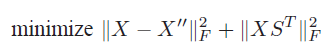# Solving a semidefinite programming (SDP) problem using the MATLAB packages CVX

Hi everybody, How can I find the unknown matrix X and solve this equation using the semidefinite programming (SDP) in CVX matlab package ?Are X’’ and S known (i.e., input data)?

How is this a semidefinite programming problem? Is there a semidefinite constraint on X?

If X’’ is some kind of derivative of X, rather than just being an input matrix, you certainly need to explain. Otherwise, whether X does or does not have a semidefinite constraint, the formulation of this problem is straightforward if you read the CVX USer’s Guide.

Hellow,
S and X-second are known and there is no constraint. This is my code, I would like to know if I implemented it correctly.

cvx_begin
variable X_hat2(NoS,Tr)
minimize( (sum(square_abs(vec(X-X_second))))+ (sum(square_abs(vec(X*S.’)))) );
cvx_end

Thank you.

It looks correct, although presuming that all matrices are real, as suggested by your variable declaration, `square` would also work instead of `square_abs`. However, per the CVX User’s Guide, it is more efficient to use `sum_square()` than `sum(square())`. You could use `sum_square(vec(X-X_second))` or `sum(sum_square((X-X_second))` or `square_pos(norm((X-X_second,'fro'))` and similarly for the other term.

1 Like